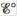# Under standard conditions, what reaction occurs, if any, when each of the following operations is performed? a. Crystals of I 2 are added to a solution of NaCl. b. Cl 2 gas is bubbled into a solution of NaI. c. A silver wire is placed in a solution of CuCl 2 d. An acidic solution of FeSO 4 is exposed to air. For the reactions that occur, write a balanced equation and calculate , ΔG °, and K at 25°C.### Chemistry: An Atoms First Approach

2nd Edition
Steven S. Zumdahl + 1 other
Publisher: Cengage Learning
ISBN: 9781305079243

#### Solutions

Chapter
Section### Chemistry: An Atoms First Approach

2nd Edition
Steven S. Zumdahl + 1 other
Publisher: Cengage Learning
ISBN: 9781305079243
Chapter 17, Problem 85E
Textbook Problem
1 views

## Under standard conditions, what reaction occurs, if any, when each of the following operations is performed?a. Crystals of I2 are added to a solution of NaCl.b. Cl2 gas is bubbled into a solution of NaI.c. A silver wire is placed in a solution of CuCl2d. An acidic solution of FeSO4 is exposed to air.For the reactions that occur, write a balanced equation and calculate,ΔG°, and K at 25°C.

(a)

Interpretation Introduction

Interpretation:

Various questions based on the displacement of one ion from its solution by other are to be answered and the E° , ΔG° and K for the possible reaction are to be calculated.

Concept introduction:

The relationship between reduction potential and standard reduction potential value and activities of species present in an electrochemical cell at a given temperature is given by the Nernst equation.

The value of Ecell is calculated using Nernst formula,

E=E°(RTnF)ln(Q)

At room temperature the above equation is specifies as,

E=E°(0.0591n)log(Q)

The relationship between cell potential and Gibbs free energy change is given by the formula,

ΔG°=nFE°cell

To determine: The reaction, if any, when crystals of I2 are added to solution of NaCl .

### Explanation of Solution

The reduction potential value for I2 is,

I2+2e2IE°=0.52V

The reduction potential value for Cl

(b)

Interpretation Introduction

Interpretation:

Various questions based on the displacement of one ion from its solution by other are to be answered and the E° , ΔG° and K for the possible reaction are to be calculated.

Concept introduction:

The relationship between reduction potential and standard reduction potential value and activities of species present in an electrochemical cell at a given temperature is given by the Nernst equation.

The value of Ecell is calculated using Nernst formula,

E=E°(RTnF)ln(Q)

At room temperature the above equation is specifies as,

E=E°(0.0591n)log(Q)

The relationship between cell potential and Gibbs free energy change is given by the formula,

ΔG°=nFE°cell

To determine: The reaction that takes place when Cl2 gas is bubbled into a solution of NaI .

(c)

c. There occurs no reaction when a silver wire is placed in a solution of CuCl2 .

Interpretation Introduction

Interpretation:

Various questions based on the displacement of one ion from its solution by other are to be answered and the E° , ΔG° and K for the possible reaction are to be calculated.

Concept introduction:

The relationship between reduction potential and standard reduction potential value and activities of species present in an electrochemical cell at a given temperature is given by the Nernst equation.

The value of Ecell is calculated using Nernst formula,

E=E°(RTnF)ln(Q)

At room temperature the above equation is specifies as,

E=E°(0.0591n)log(Q)

The relationship between cell potential and Gibbs free energy change is given by the formula,

ΔG°=nFE°cell

To determine: The reaction, if any, when a silver wire is placed into a solution of CuCl2

(d)

Interpretation Introduction

Interpretation:

Various questions based on the displacement of one ion from its solution by other are to be answered and the E° , ΔG° and K for the possible reaction are to be calculated.

Concept introduction:

The relationship between reduction potential and standard reduction potential value and activities of species present in an electrochemical cell at a given temperature is given by the Nernst equation.

The value of Ecell is calculated using Nernst formula,

E=E°(RTnF)ln(Q)

At room temperature the above equation is specifies as,

E=E°(0.0591n)log(Q)

The relationship between cell potential and Gibbs free energy change is given by the formula,

ΔG°=nFE°cell

To determine: The reaction, if any, an acidic solution of FeSO4 is exposed to air.

### Still sussing out bartleby?

Check out a sample textbook solution.

See a sample solution

#### The Solution to Your Study Problems

Bartleby provides explanations to thousands of textbook problems written by our experts, many with advanced degrees!

Get Started

Find more solutions based on key concepts
What is the length of the nail reported to the correct number of significant figures?

General Chemistry - Standalone book (MindTap Course List)

To temporarily increase the bodys water content, a person need only. a. consume extra salt. b. consume extra su...

Nutrition: Concepts and Controversies - Standalone book (MindTap Course List)

Which of Earths internal zones is the largest?

Fundamentals of Physical Geography

When an aluminum bar is connected between a hot reservoir at 725 k and a cold reservoir at 310 K, 2.50 kJ of en...

Physics for Scientists and Engineers, Technology Update (No access codes included)

What are the evolutionary advantages of possessing vertebrae?

Oceanography: An Invitation To Marine Science, Loose-leaf Versin